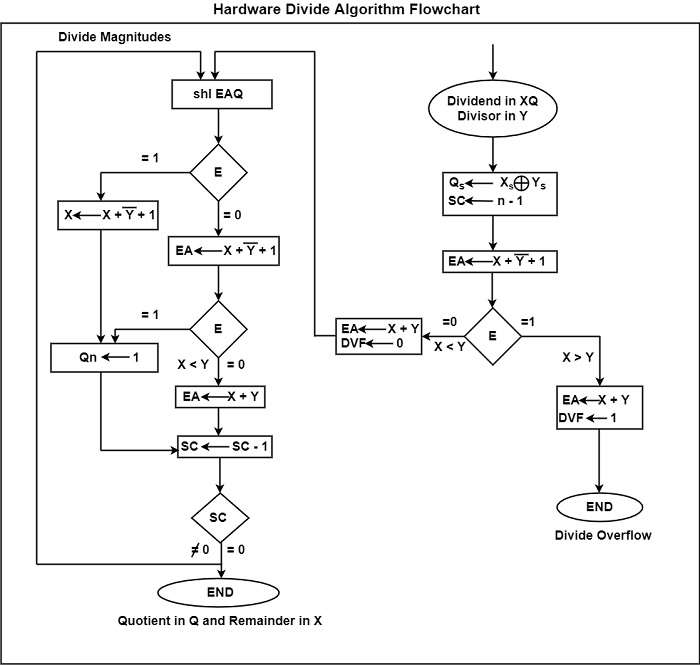# What is the division of binary numbers in Computer Architecture?

The binary division is similar to division in decimals. The process involves successive comparison, shifting, and subtraction. The division of binary numbers is easy compared to the division of decimal numbers because the quotient is either 0 or 1. It is also not necessary to check the number of times the dividend (partial remainder) fits into the divisor.

## Divide Overflow

In a computer system, the division operation can lead to a quotient with an overflow because the registers cannot hold a number that exceeds the standard length. To understand this better, consider a system with a standard 5-bit register.

One register is used to hold the divisor and the other to hold the dividend. In case the quotient consists of 6 bits, 5 bits of the quotient will be stored in a 5-bit register. Therefore, the overflow bit needs a flip-flop to store the sixth bit.

The divide overflow condition occurs in case the high-order half bits of the dividend comprises a number that is greater than or equal to the divisor. One other point that needs to be considered in the division is that it is advisable to avoid division by zero. The overflow condition is generally detected when a flip-flop is set. This flip-flop is known as DVF.

## Hardware Algorithm

The hardware divide algorithm can be interpreted easily with the help of a figure. The figure shows the hardware divide algorithm using a flow chart.The dividend is in X and Q and the divisor is in Y. The sign of the resultant is stored in , which is a part of the quotient. A constant is set into the SC to determine the multiple bits in the quotient.

The testing of the divide overflow condition takes place by subtracting the divisor present in Y from half of the bits of the dividend that is stored in X.

• In the case of XY, the DVF is set and an untimely cancellation of the operation takes place.
• In case X < Y, the divide overflow does not occur. Therefore, the value of the dividend is restored by adding Y to X.

The division of the magnitudes begins by transferring the dividend present in XQ to the left and by transferring the high-order bit to E. In the case of the bit transferred into E is 1, EA > Y because EA includes a 1 followed by n - 1 bit and Y includes just n - 1 bit.

Therefore, Y is subtracted from EA, and 1 is placed in QS for the quotient bit. Because Register X does not have the high-order bit of the dividend, its value is EA - 2n−1. If the 2’s complement of Y is added to this value, the output would be as follows −

(EA - 2n−1) + ( 22n−1 − Y) = EA − Y

In case E should remain as 1, the carry from the addition should not be transferred to E.

In case the shift left operation inserts 0 into E, the divisor is subtracted by inserting its 2’s complement value. The carry has to be transferred to E. In case E = 1, it means that X Y and as such, QS is set to 1. In case E = 0, it means that X < Y, and therefore, the original number is restored by adding Y to X. In this case, the 0 that was inserted during a shift is left in QS.